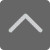# 小学口算题大全：二年级100以内加减法口算题(二)

2018-11-02 16:31:46 　来源：网络 文章作者：网络
 免费下载 3个G小报手抄报电子版 小学1-5年级上册期末复习资料 [ 戳我免费下载 ]

91-75= 25+26+4= 72-46= 16+27=

38+37+13= 28+62= 39+32= 45+15+15=

18+25= 48-4= 34+5+5= 16+72=

80-54= 13+16+54= 44+16= 24+18=

54+21+9= 42+29= 42+16= 17+28+28=

13+41= 23+11= 55+31+9= 73-71=加入QQ群，与更多家长交流经验！
2
 小学交流群：639215153 初中交流群：551802726 高中交流群：591337342 上海幼儿园升小学家长学习交流群：772707735